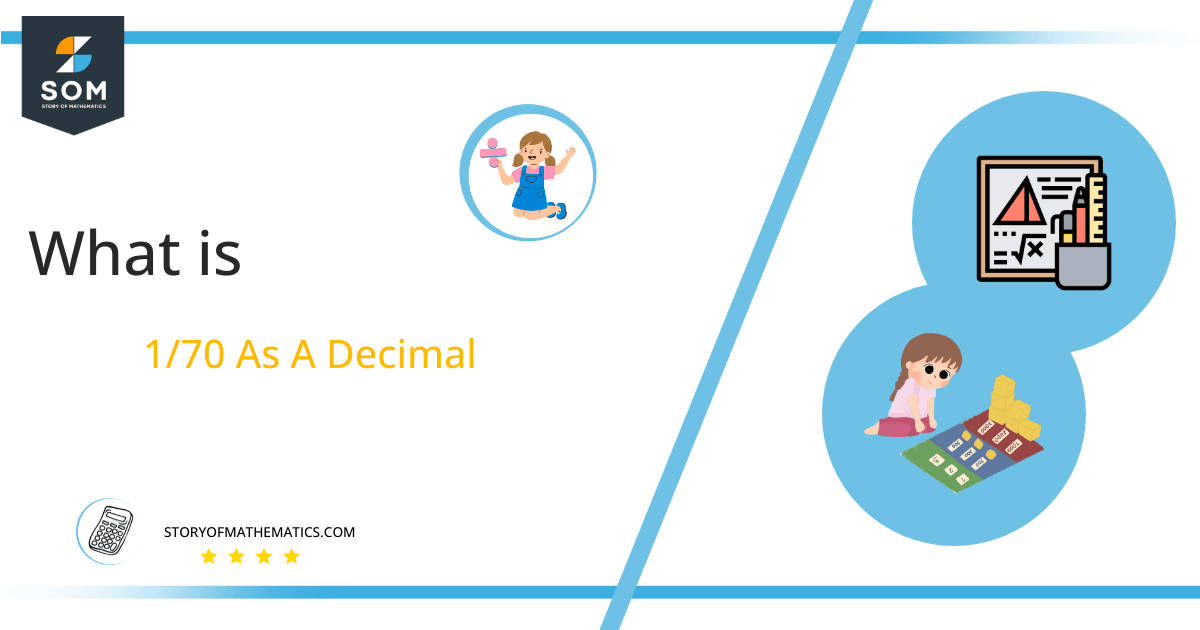# What Is 1/70 as a Decimal + Solution With Free Steps

The fraction 1/70 as a decimal is equal to 0.01428571.

A fraction is a mathematical expression that is written in p/q form where p and q are the whole numbers and q≠0.  In fractions, p and q are the numerators and denominators respectively. Simplifying through the long division method fraction values obtained, are always positives that can be decimal values or whole numbers.

Here, we are more interested in the division types that result in a Decimal value, as this can be expressed as a Fraction. We see fractions as a way of showing two numbers having the operation of Division between them that result in a value that lies between two Integers.Now, we introduce the method used to solve said fraction to decimal conversion, called Long Division, which we will discuss in detail moving forward. So, let’s go through the Solution of fraction 1/70.

## Solution

First, we convert the fraction components, i.e., the numerator and the denominator, and transform them into the division constituents, i.e., the Dividend and the Divisor, respectively.

This can be done as follows:

Dividend = 1

Divisor = 70

We introduce the most important quantity in our division process: the Quotient. The value represents the Solution to our division and can be expressed as having the following relationship with the Division constituents:

Quotient = Dividend $\div$ Divisor = 1 $\div$ 70

This is when we go through the Long Division solution to our problem. The following figure shows the long division:Figure 1

## 1/70 Long Division Method

We start solving a problem using the Long Division Method by first taking apart the division’s components and comparing them. As we have 1 and 70, we can see how 1 is Smaller than 70, and to solve this division, we require that 1 be Bigger than 70.

This is done by multiplying the dividend by 10 and checking whether it is bigger than the divisor or not. If so, we calculate the Multiple of the divisor closest to the dividend and subtract it from the Dividend. This produces the Remainder, which we then use as the dividend later.

Now, we begin solving for our dividend 1, which after getting multiplied by 10 twice and adding zero in the Quotient after the decimal point becomes 100.

We take this 100 and divide it by 70; this can be done as follows:

100 $\div$ 70 $\approx$ 1

Where:

70 x 1 = 70

This will lead to the generation of a Remainder equal to 100 – 70 = 30. Now this means we have to repeat the process by Converting the 30 into 300 and solving for that:

300 $\div$ 70 $\approx$ 4

Where:

70 x 4 = 280

Therefore,  Remainder equals to 300 – 280 = 20. Now we stop solving this problem, we have a Quotient generated after combining the two pieces of it as 0.014=z, with a Remainder equal to 20.Images/mathematical drawings are created with GeoGebra.